# Asymptotic Matching

Let us assume that the helical magnetic island chain possesses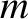periods in the poloidal direction, and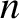periods in the toroidal direction. Let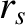be the minor radius of the rational surface.

Let us reuse the analysis of Sections 3.17, 7.2, and 9.4. In the absence of a wall (i.e.,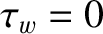), but in the presence of a static magnetic perturbation generated by a steady helical current,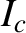, withperiods in the poloidal direction, andperiods in the toroidal direction, running though an external magnetic field-coil located outside the plasma at minor radius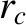, we find that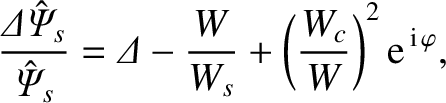(13.1)

where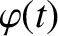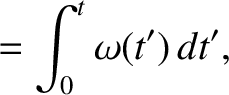(13.2)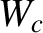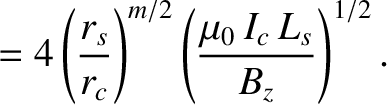(13.3)

Here,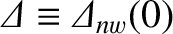is the (real dimensionless) no-wall tearing stability index evaluated at zero island width [see Equation (7.4)],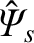the normalized reconnected helical magnetic flux at the rational surface [see Equation (3.184)],the normalized helical sheet current density at the rational surface [see Equation (3.183)],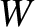the full radial width of the magnetic island chain [see Equation (5.129)],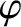the helical phase of the island chain measured with respect to that of the static resonant magnetic perturbation,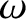the instantaneous island rotation frequency in the laboratory frame [see Equation (3.189)],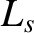the magnetic shear-length at the rational surface [see Equation (5.27)], and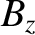the equilibrium toroidal magnetic field-strength. Moreover,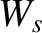is specified in Equation (12.2), andis a measure of the width of the locked magnetic island chain that the resonant magnetic perturbation would induce at the rational surface in the absence of localized shielding currents. Finally, we have made the simplifying approximation that the equilibrium plasma current external to the rational surface is negligible. (See Section 7.2.)